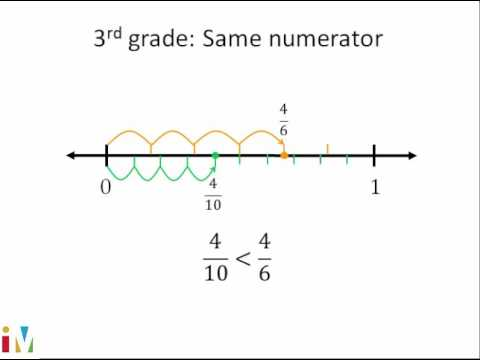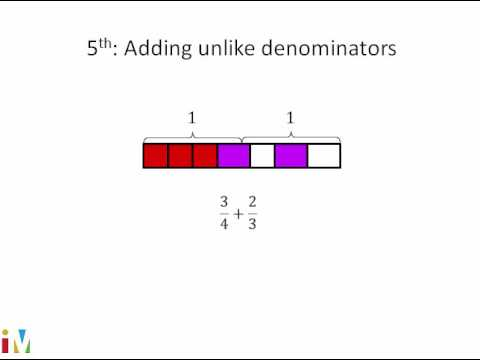Video

# Multiplying 2 fractions: number line (Full video)

Description: This video uses a number line to explain multiplying a unit fraction by a non-unit fraction. In a previous video, we saw that we could view 2/3 times 6 as whatever number is 2/3 of the way to 6 on the number line, which we saw is 4. Now, what we want to do now is apply that same idea, but to multiply not a fraction times a whole number, but a fraction times a fraction. So let's say that we wanted to take 3/4 and multiply it by 1/2.

### Other videos you might be interested in### Comparing Fractions

#### Illustrative Mathematics